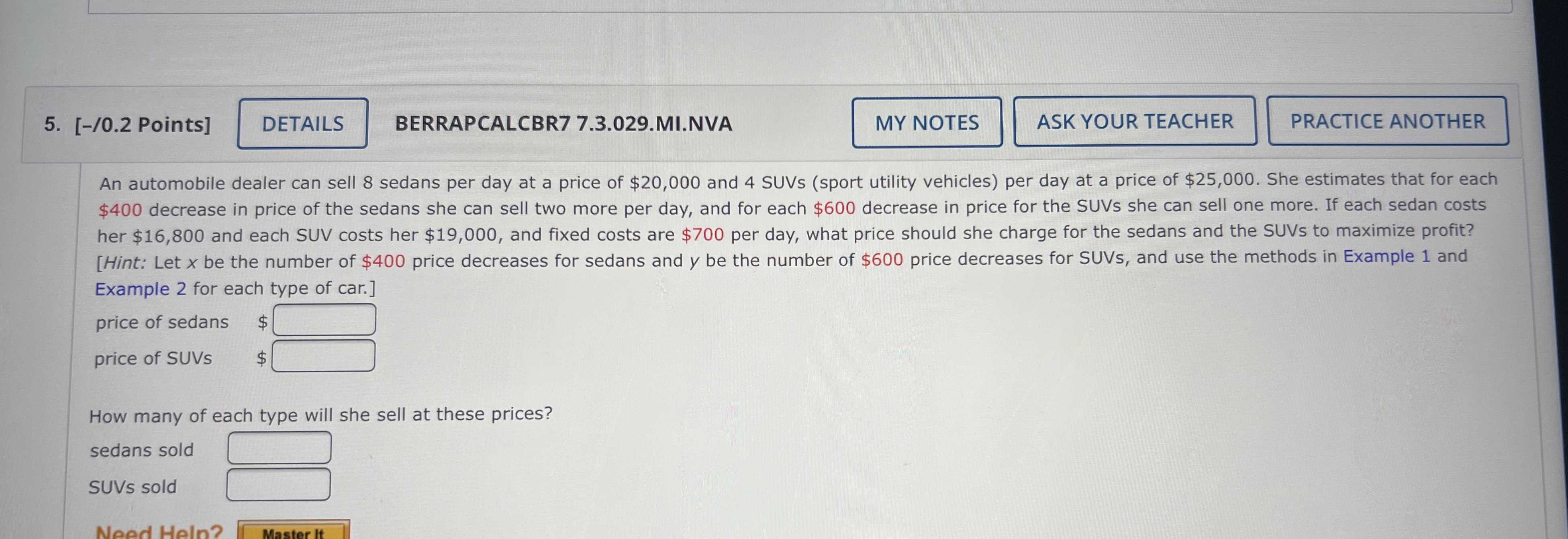### Still have math questions?

Algebra
QuestionAn automobile dealer can sell $$8$$ sedans per day at a price of $$\ 20,000$$ and $$4$$ SUVs (sport utility vehicles) per day at a price of $$\ 25,000$$ . She estimates that for each

$$\ 400$$ decrease in price of the sedans she can sell two more per day, and for each $$\ 600$$ decrease in price for the SUVs she can sell one more. If each sedan costs her $$\ 16,800$$ and each SUV costs her $$\ 19,000$$ , and fixed costs are $$\ 700$$ per day, what price should she charge for the sedans and the SUVs to maximize profit?

price of sedans

price of SUVs

How many of each type will she sell at these prices?

sedans sold SUVs sold